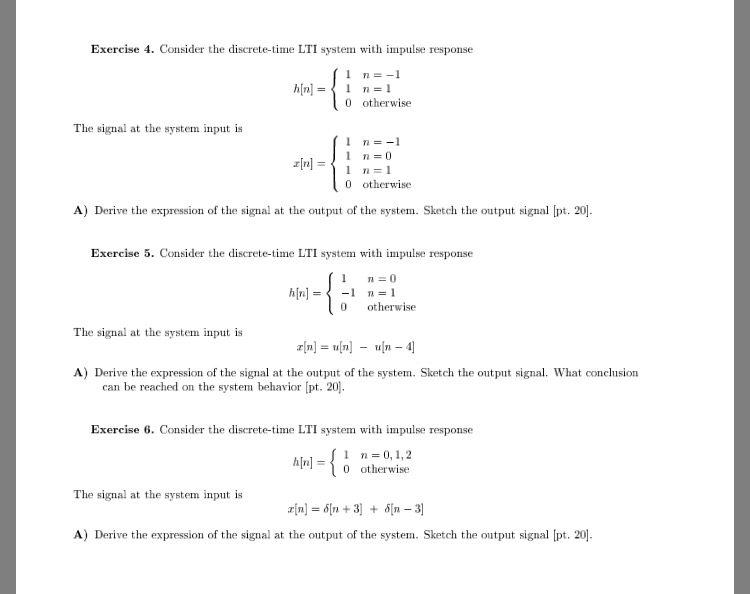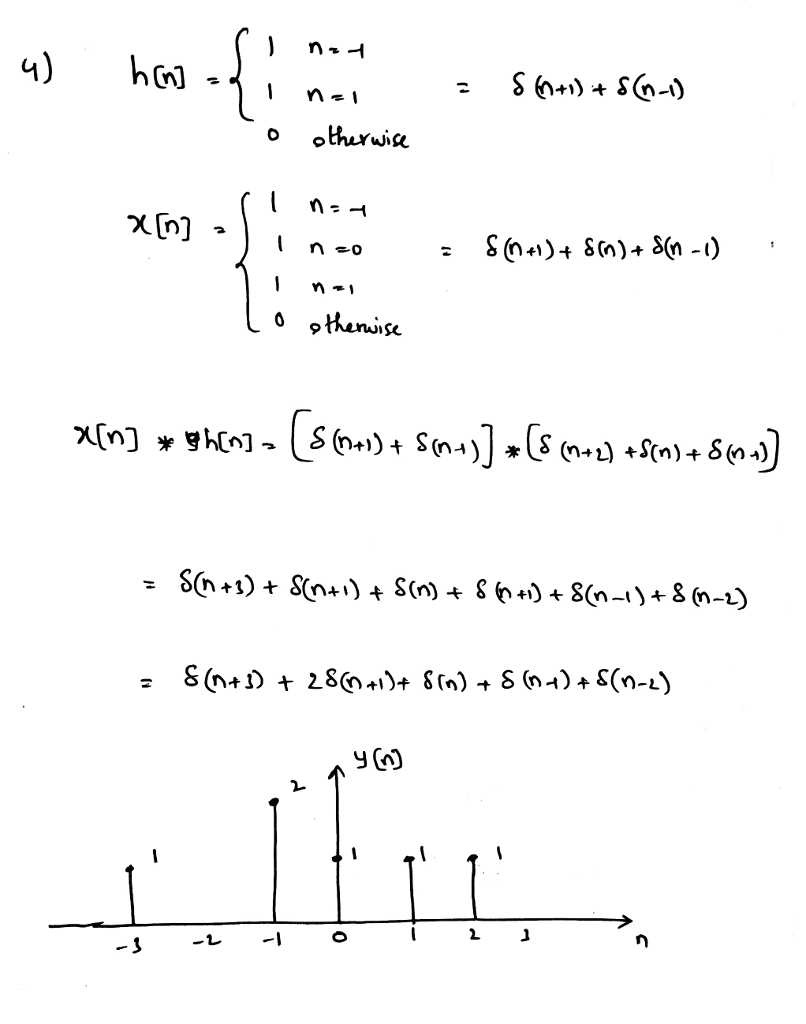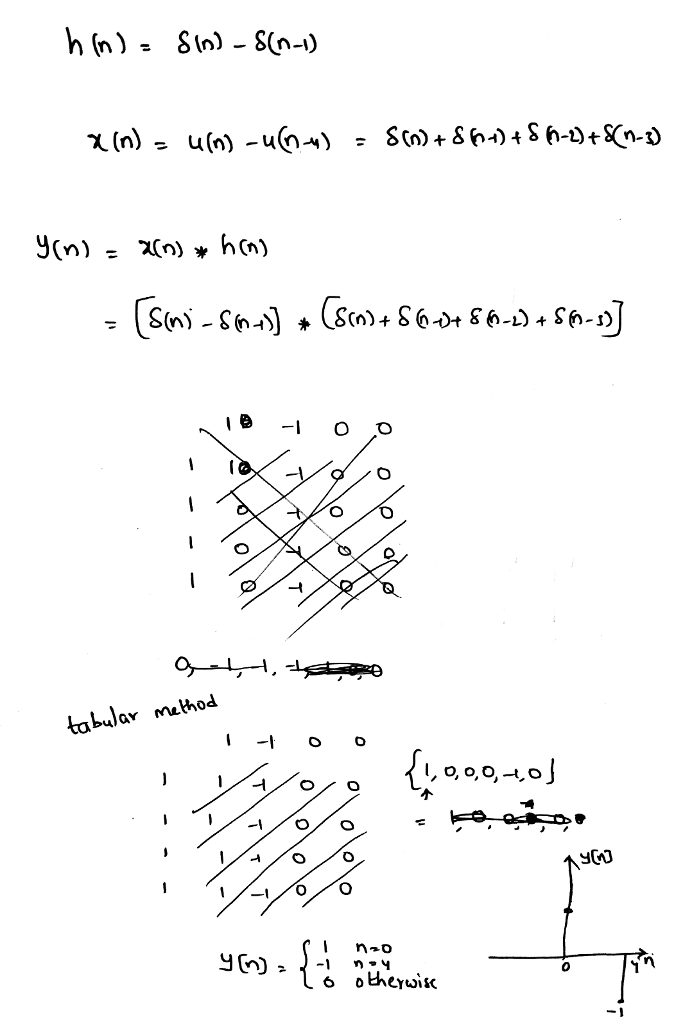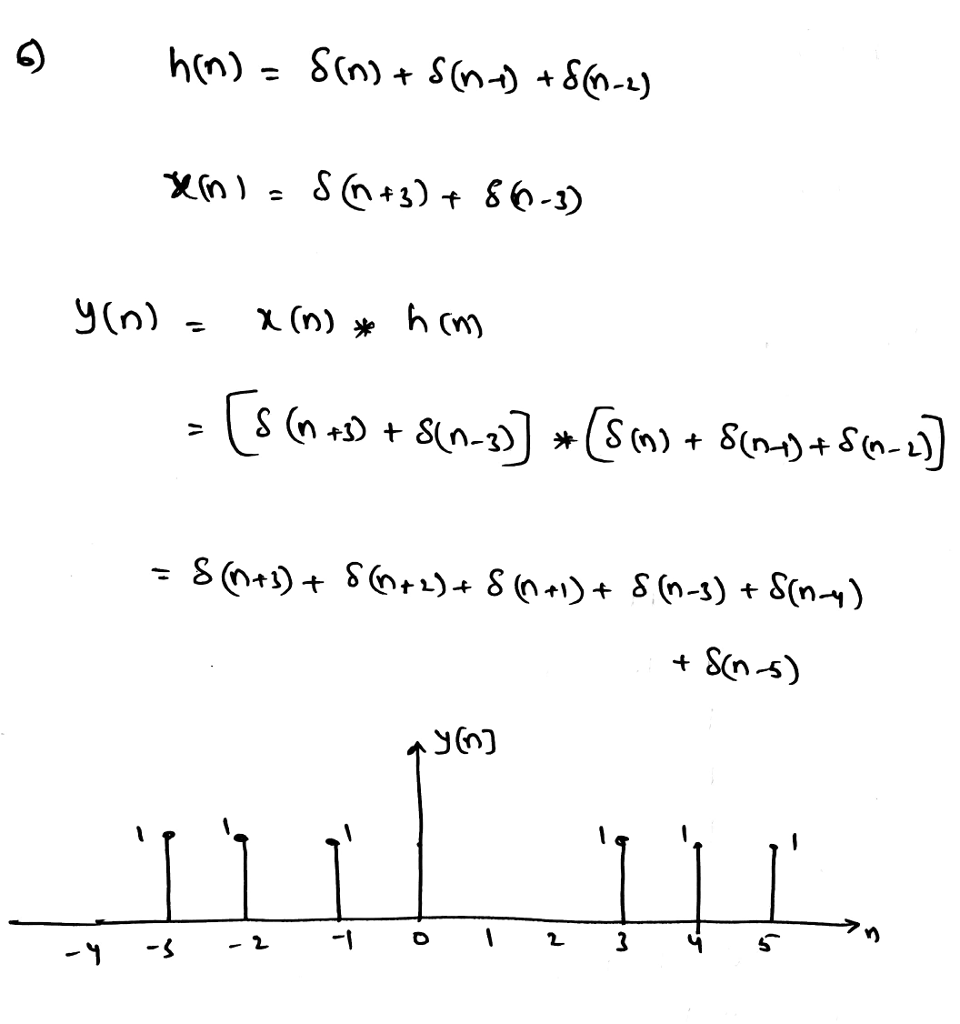# Homework Solution: otherwise Derive the expression of the signal at the output of the system. Sketch the o…Consider the discrete-time LTI system with impulse response h[n] = {1 n = -1 1 n = 1 0 otherwise The signal at the system input is x[n] = {1 n = -1 1 n = 0 1 n = 1 0 otherwise Derive the expression of the signal at the output of the system. Sketch the output signal. Consider the discrete-time LTI system with impulse response h[n] = {1 n 0 -1 n = 1 0 otherwise The signal at the system input is x[n] = u[n] - u[n - 4] Derive the expression of the signal at the output of the system. Sketch the output signal. What conclusion can be reached on the system behavior. Consider the discrete-time LTI system with impulse response h[n] = {1 n = 0, 1, 2 0 otherwise The signal at the system input is x[n] = delta[n + 3] + delta[n - 3] Derive the expression of the signal at the output of the system. Sketch the output signal.